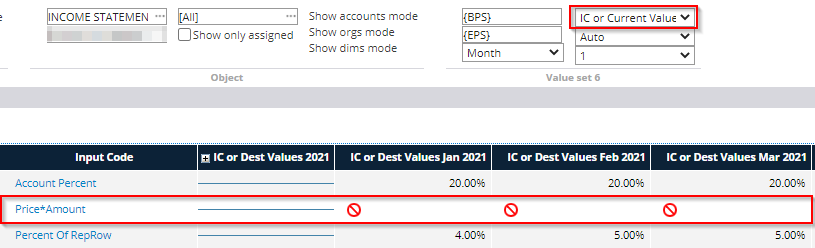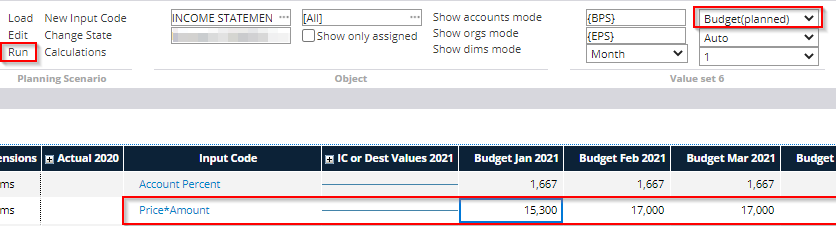The Price * Amount input code is used to obtain a value for the selected account by entering separately the price and the amount. Input code multiplies the price by the amount.

Input Code Properties -tab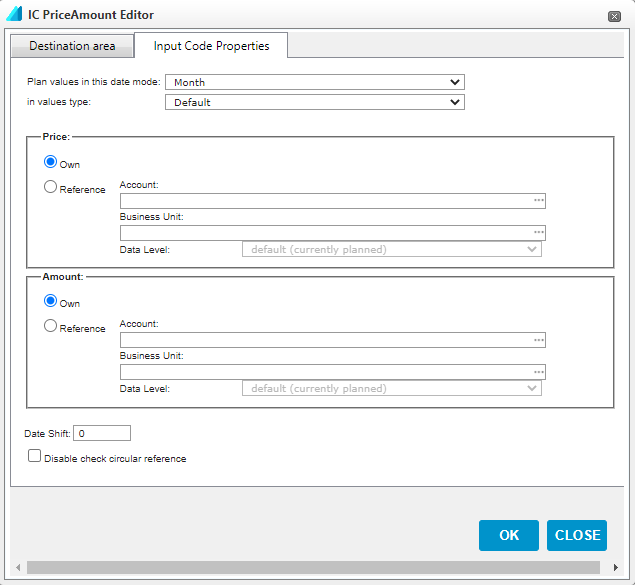Plan values in this date mode: This setting determines the accuracy level at which the calculation is performed. With monthly accuracy, a separate percentage is determined for each month, while, for example, with annual accuracy, only one percentage value is determined for the entire year.

In values type: Specifies the type of calculated values stored in the system. In most cases, you can use the default setting, in which case the type of values calculated depends on the settings made on the chart of accounts.

Own: Specifies the price / quantity input method. This option used if the price or quantity are entered manually on the input form.

Reference: Specifies the input method for the price or amount. This option is used if the price or amount is calculated from auxiliary accounts.

Date Shift: A negative value means that the values of the previous month are used as input data, while a positive value means that the percentage is calculated from the values of the following year.

Disable check circular reference: Allows the use of circular references in the input code, i.e. references that depend on the results of the same formula.

Destination area -tab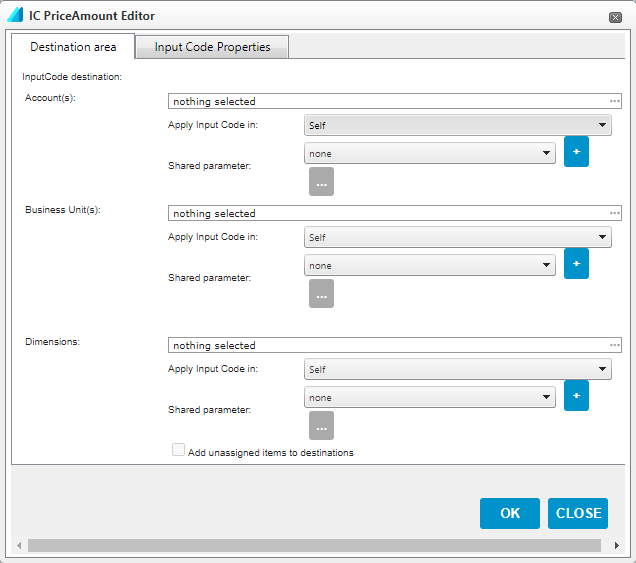Account(s): Select the accounts / account groups for which the input code is used

Business Unit(s): The organizational levels at which the input code is used

Dimensions(s): Dimensions or dimension objects for which the input code is used. By selecting 'Add unassigned items to destinations', the calculation is also performed for values that are not dimensioned

For all of the above parameters, there are two choices to determine how extensively the calculation is performed:

Apply Input Code in: Specifies whether the input code is used only at the selected level, its cost centers, or parallel levels

Shared parameter: A common parameter that contains user-defined organizational levels or accounts  can be selected in this field. This function can be used if you want to add several different input codes to the same set of accounts or organizations, without defining them in each input code separately. A new common parameter is created from the + sign.

Once all the above parameters have been defined, select 'OK' to create the input code.

Option 1: Entering the price and amount on the input template

If 'Own' has been selected as the price and / or amount input method on the input code properties tab, their values must be entered manually on the input form. The input value can only be entered in the IC or Current Values mode, which must be selected as shown.

The values are entered either in the year column or for individual months, depending on the plan date mode of the input values in the parameters.

In the example, the planning accuracy is set to month, in which case the price and quantity are entered for each month separately. The year column has a blue line to indicate that the value cannot be entered on an annual basis.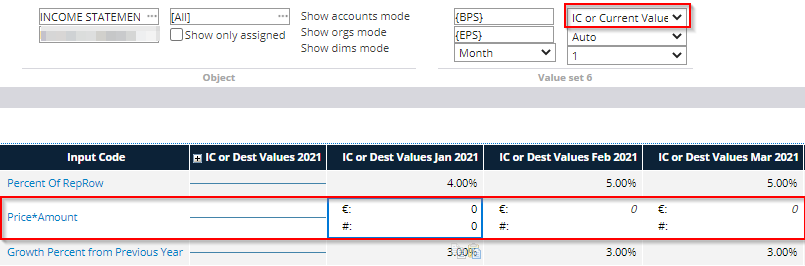The value is entered by double-clicking on a cell with a € sign in either the month or year column (depending on which selection has been used as plan date mode), which opens a dialog box where you can enter the price and / or quantity of that cell.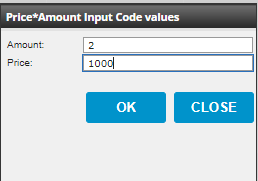Once the percentages have been entered, the actual calculation for the input form is performed by selecting 'Run' from the toolbar.

The values calculated with the input code can be viewed by selecting Budget or Planned as the display mode, depending on whether the input form is for Budget or Forecast.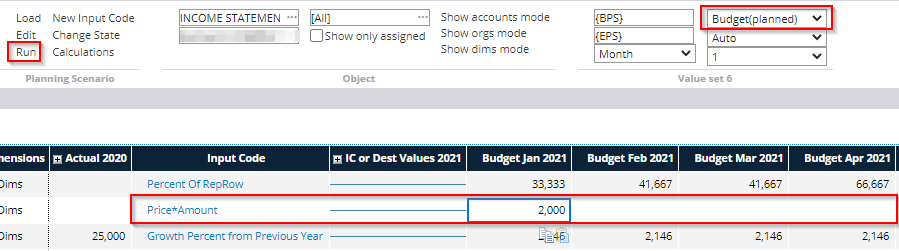Option 2: Calculating the price and amount from the auxiliary accounts

If the price and / or amount input method is set to Reference on the input code properties tab, the values of these are retrieved from the auxiliary accounts. This is done by selecting the desired account from the chart of accounts in the Account field on the Input Code Properties tab and the organizational level whose balance is used in the calculation in the Unit field. Data level field can be set to Default if the output data is calculated from the same data level as the input base.

In this case, it is no longer possible to manually enter values into the input forms, as the values are calculated from other accounts, such as in this example from the lines Quantity and Price, to which output values can be entered.